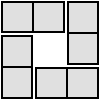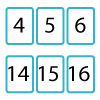#### You may also like### 4 Dom

Use these four dominoes to make a square that has the same number of dots on each side.### Twenty Divided Into Six

Katie had a pack of 20 cards numbered from 1 to 20. She arranged the cards into 6 unequal piles where each pile added to the same total. What was the total and how could this be done?### Mystery Matrix

Can you fill in this table square? The numbers 2 -12 were used to generate it with just one number used twice.

# Satisfying Four Statements

##### Age 7 to 11Challenge Level

Ash, Si, Sami and Mani are playing a game.
Each of them writes down a statement that describes a set of numbers.

Ash writes "Multiples of five".
Si writes "Triangular numbers".
Sami writes "Even, but not multiples of four".
Mani writes "Multiples of three but not multiples of nine".

Can you find some two-digit numbers that belong in two of the sets?
Can you find some two-digit numbers that belong in three sets?
What is the smallest number that belongs in all four sets?

How could you describe the pattern of the numbers that satisfy both Ash's and Sami's statements?
How about the numbers that satisfy both Ash's and Mani's statements?

Can you describe patterns for other pairs of statements?

You might now like to play the game Statement Snap and then have a go at Charlie's Delightful Machine.